• 高斯差分滤波结果 原图像 高斯差分滤波结果 Show me the code #include <iostream> #include <string> #include <opencv2/imgproc/imgproc.hpp> #include <opencv2/highgui.hpp> using ...
高斯差分滤波结果
原图像高斯差分滤波结果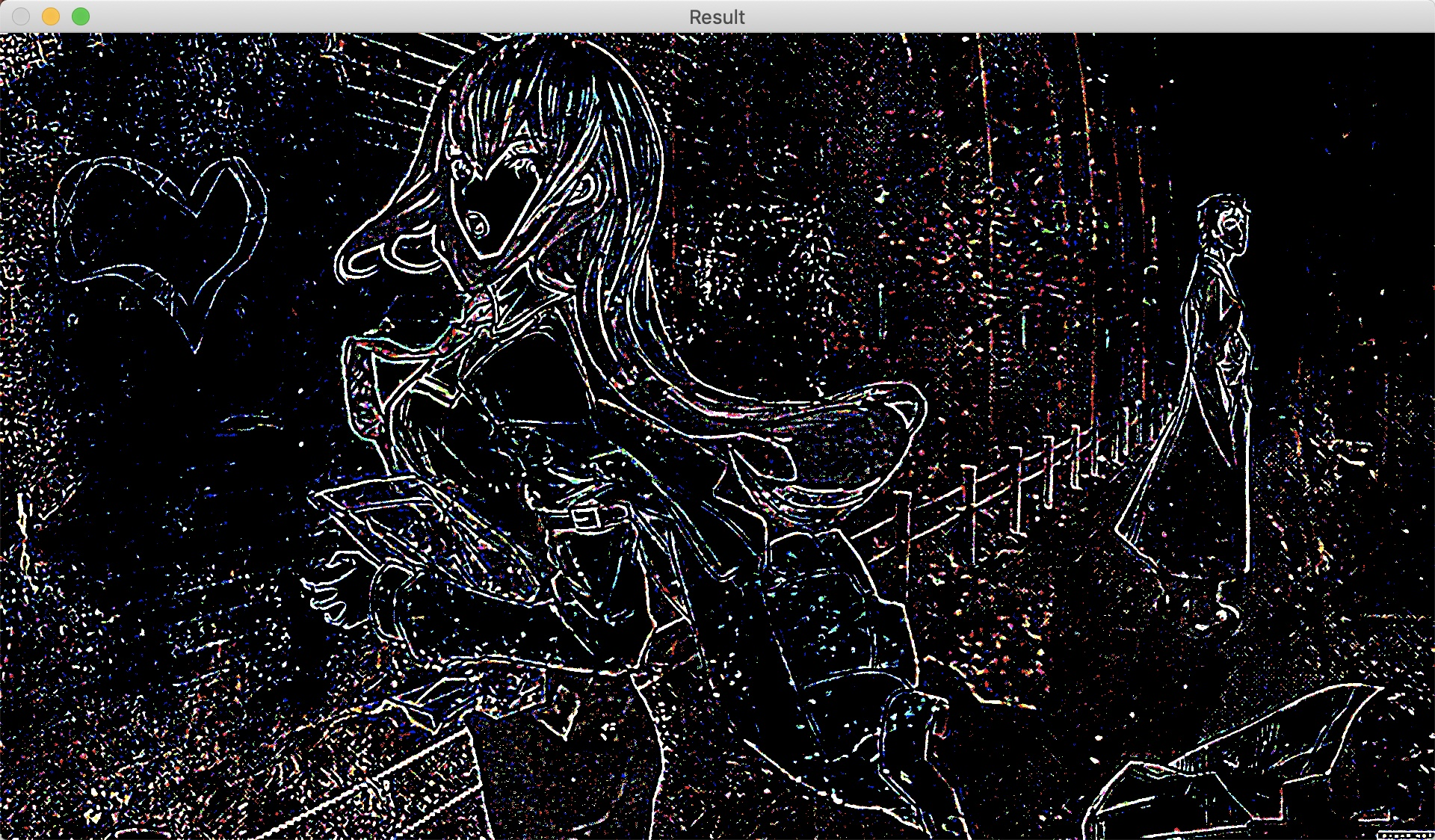Show me the code
#include <iostream>
#include <string>
#include <opencv2/imgproc/imgproc.hpp>
#include <opencv2/highgui.hpp>

using namespace std;

int main() {
// 图像路径
const string fileName = "xxx.jpg";

// 读取图像

// 创建差分存放结果及中间图像空间
cv::Mat pro1, pro2, result;

// 设置两次高斯滤波的sigma
const float sigma1 = 1.6;
const float sigma2 = 2.0;

// 两次不同sigma高斯滤波
cv::GaussianBlur(origin,
pro1,
cv::Size(0, 0),
sigma1);

cv::GaussianBlur(origin,
pro2,
cv::Size(0, 0),
sigma2);

// 高斯滤波结果差分
result = pro1 - pro2;

// 显示图像
cv::imshow("Origin", origin);
cv::imshow("Result", result * 255);

cv::waitKey(0);
}


展开全文差分滤波
• 其中L_FaceImg_gmma是经过伽马光线校正之后的图像，分别作滤波，之后差分相减，就得到高斯差分滤波的结果了……囧。明天去pudn上查查代码，看看是不是这样写的…… 下面是fspecial函数的使用样例，写上来原因是蛮...

自己调研之后，写的伽马校正：
TestImgNum =1 ;00 335
str = strcat('.\',num2str(TestImgNum),'.bmp');
figure,imshow(uint8(L_FaceImg));
temp = double(L_FaceImg);
temp = temp./256;
gamma = 1/2.2;
% y=gamma(temp);%实现gamma校正
% y = gamma_filter(temp,gamma);
y = y.*256;
figure,imshow(uint8(y));
xb调研完之后，写的代码：
L_FaceImg=double(L_FaceImg_org);
%L_FaceImg=L_FaceImg.^0.2;
gama=2;
p=255/255^(gama);p=(1/p)^(1/gama);
for i=1:imrow
for
j=1:imcol
%for l=1:k
% r(i,j,l)=floor(p*double(I(i,j,l))^(1/gama));
L_FaceImg_gmma(i,j)=floor(p*L_FaceImg(i,j)^(1/gama));
%end
end
end
完全是原理性的东西。
高斯查分滤波，在网上查DoG查了好久，最后终于终于在pudn上面找到相关c代码，结果被xb两句话解决了……具体见下面：
low=
imfilter(L_FaceImg_gmma,fspecial('gaussian',7,1),'same','replicate');
lowlow=imfilter(L_FaceImg_gmma,fspecial('gaussian',9,2),'same','replicate');
Resimage=low-lowlow;
其中L_FaceImg_gmma是经过伽马光线校正之后的图像，分别作滤波，之后差分相减，就得到高斯差分滤波的结果了……囧。明天去pudn上查查代码，看看是不是这样写的……
下面是fspecial函数的使用样例，写上来原因是蛮喜欢subplot函数，哇咔咔~
subplot(2,2,1);imshow(I);title('Original Image');
H = fspecial('motion',20,45);
MotionBlur = imfilter(I,H,'replicate');%replicate表示Input array
values outside the bounds of the array are
assumed to equal the nearest array border value
输入值自动对齐到最近邻
subplot(2,2,2);imshow(MotionBlur);title('Motion Blurred
Image');
H = fspecial('disk',10);
blurred = imfilter(I,H,'replicate');
subplot(2,2,3);imshow(blurred);title('Blurred Image');
H = fspecial('unsharp');
sharpened = imfilter(I,H,'replicate');
subplot(2,2,4);imshow(sharpened);title('Sharpened Image');
对比均衡化还没搞明白，先把代码贴出来，供慢慢研究……
%Contrast Equalization.
a=0.1;
for i=1:imrow
for j=1:imcol
Resimage_temp(i,j)=(abs(Resimage(i,j)))^a; end
end
mu=mean(mean(Resimage_temp));
Resimage=Resimage./(mu^(1/a));
tt=10;
for i=1:imrow
for j=1:imcol
Resimage_temp(i,j)=min(tt,(abs(Resimage(i,j)))^a); end
end
mu=mean(mean(Resimage_temp));
Resimage=Resimage./(mu^(1/a));
Resimage=tt*tanh(Resimage/tt);
Resimage_max=max(max(Resimage));
Resimage_min=min(min(Resimage));
for i=1:imrow
for j=1:imcol
Resimage(i,j)=(Resimage(i,j)-Resimage_min)/(Resimage_max-Resimage_min)*255; end
end
% imshow(uint8(Resimage));
%提取LBP模板
% [result , Lbpface] = lbp(L_FaceImg);
%figure,imshow(uint8(Lbpface));
L_FaceImg_bandpass=uint8(Resimage);
TestImage
= strcat('.\M00模糊脸_bandpass\',num2str(ii),'.bmp');
imwrite(uint8(L_FaceImg_bandpass),TestImage,'bmp');
% figure,imshow(uint8(blurred));
end
晕死，就俩公式：其中a = 0.1，t= 10.看着挺简单，我咋就做不出来呢？
真是的啊我

展开全文• 其中L_FaceImg_gmma是经过伽马光线校正之后的图像，分别作滤波，之后差分相减，就得到高斯差分滤波的结果了……囧。明天去pudn上查查代码，看看是不是这样写的…… 下面是fspecial函数的使用样例，写上来原因是...
 http://blog.sina.com.cn/s/blog_48e673350100vcg5.html
自己调研之后，写的伽马校正：
TestImgNum =1  ;00 335 str = strcat('.\',num2str(TestImgNum),'.bmp'); L_FaceImg = imread(str); figure,imshow(uint8(L_FaceImg)); temp = double(L_FaceImg); temp = temp./256; gamma = 1/2.2;  y = imadjust(temp,[0; 1],[0; 1],gamma) % y=gamma(temp);%实现gamma校正 %  y = gamma_filter(temp,gamma); y = y.*256; figure,imshow(uint8(y));

xb调研完之后，写的代码：
L_FaceImg=double(L_FaceImg_org); %L_FaceImg=L_FaceImg.^0.2; gama=2; p=255/255^(gama);p=(1/p)^(1/gama); for i=1:imrow     for j=1:imcol         %for l=1:k        % r(i,j,l)=floor(p*double(I(i,j,l))^(1/gama));         L_FaceImg_gmma(i,j)=floor(p*L_FaceImg(i,j)^(1/gama));         %end     end end 完全是原理性的东西。
高斯查分滤波，在网上查DoG查了好久，最后终于终于在pudn上面找到相关c代码，结果被xb两句话解决了……具体见下面：
low= imfilter(L_FaceImg_gmma,fspecial('gaussian',7,1),'same','replicate'); lowlow=imfilter(L_FaceImg_gmma,fspecial('gaussian',9,2),'same','replicate');
Resimage=low-lowlow; 其中L_FaceImg_gmma是经过伽马光线校正之后的图像，分别作滤波，之后差分相减，就得到高斯差分滤波的结果了……囧。明天去pudn上查查代码，看看是不是这样写的……
下面是fspecial函数的使用样例，写上来原因是蛮喜欢subplot函数，哇咔咔~
I = imread('cameraman.tif');        subplot(2,2,1);imshow(I);title('Original Image');        H = fspecial('motion',20,45);        MotionBlur = imfilter(I,H,'replicate');%replicate表示Input array values outside the bounds of the array  are assumed to equal the nearest array border value 输入值自动对齐到最近邻        subplot(2,2,2);imshow(MotionBlur);title('Motion Blurred Image');        H = fspecial('disk',10);        blurred = imfilter(I,H,'replicate');        subplot(2,2,3);imshow(blurred);title('Blurred Image');        H = fspecial('unsharp');        sharpened = imfilter(I,H,'replicate');        subplot(2,2,4);imshow(sharpened);title('Sharpened Image');

对比均衡化还没搞明白，先把代码贴出来，供慢慢研究……
%Contrast Equalization. a=0.1; for i=1:imrow        for j=1:imcol        Resimage_temp(i,j)=(abs(Resimage(i,j)))^a;        end end  mu=mean(mean(Resimage_temp));  Resimage=Resimage./(mu^(1/a));
tt=10; for i=1:imrow        for j=1:imcol        Resimage_temp(i,j)=min(tt,(abs(Resimage(i,j)))^a);        end end mu=mean(mean(Resimage_temp)); Resimage=Resimage./(mu^(1/a)); Resimage=tt*tanh(Resimage/tt); Resimage_max=max(max(Resimage)); Resimage_min=min(min(Resimage));  for i=1:imrow        for j=1:imcol        Resimage(i,j)=(Resimage(i,j)-Resimage_min)/(Resimage_max-Resimage_min)*255;        end  end % imshow(uint8(Resimage));    %提取LBP模板 % [result , Lbpface] = lbp(L_FaceImg);  %figure,imshow(uint8(Lbpface)); L_FaceImg_bandpass=uint8(Resimage);
TestImage = strcat('.\M00模糊脸_bandpass\',num2str(ii),'.bmp');     imwrite(uint8(L_FaceImg_bandpass),TestImage,'bmp'); %     figure,imshow(uint8(blurred)); end

晕死，就俩公式：其中a = 0.1，t= 10.看着挺简单，我咋就做不出来呢？
真是的啊我
展开全文• http://blog.csdn.net/yangyangyang20092010/article/details/48576007  也对高斯权值做了详细的解释 ...高斯滤波是一种线性平滑滤波，适用于消除高斯噪声，在图像处理的降噪、平滑中应用较多，特
转载地址  http://blog.csdn.net/dcrmg/article/details/52304446#reply
http://blog.csdn.net/yangyangyang20092010/article/details/48576007  也对高斯权值做了详细的解释

高斯滤波是一种线性平滑滤波，适用于消除高斯噪声，在图像处理的降噪、平滑中应用较多，特别是对抑制或消除服从正态分布的噪声非常有效。
高斯滤波的过程其实就是对整幅图像进行加权平均操作的过程。滤波后图像上每一个像素的灰度值大小，由其本身和邻域内的其他像素共同决定。具体实现是：用一个大小为（2*N+1）的模板（或称卷积核、掩模）依次扫描图像中的每一个像素，用模板确定的邻域内像素的加权平均灰度替代模板中心像素点的灰度值。

一维、二维高斯分布

一维高斯函数表述为：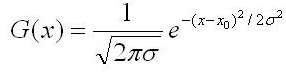对应图形：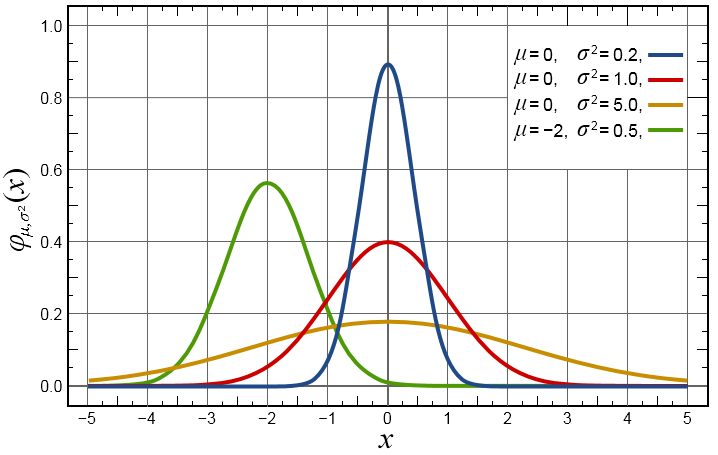二维高斯函数表述为：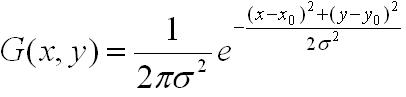对应图形：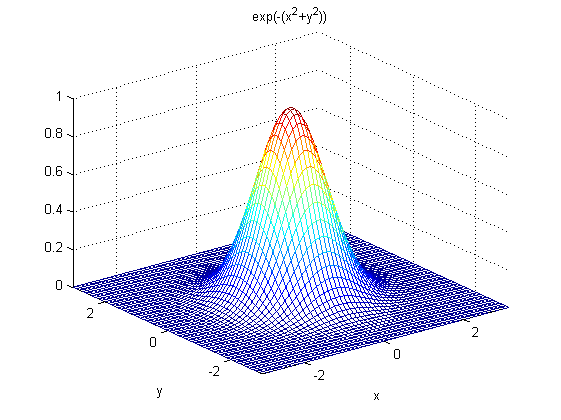一些重要特性说明：
1. 一维二维高斯函数中μ是服从正态分布的随机变量的均值，称为期望或均值影响正态分布的位置，实际的图像处理应用中一般取μ=0；σ是标准差，σ^2是随机变量的方差，σ定义了正态分布数据的离散程度，σ越大，数据分布越分散，σ越小，数据分布越集中。
在图形或滤波效果上表现为：σ越大，曲线越扁平，高斯滤波器的频带就越宽，平滑程度就越好，σ越小，曲线越瘦高，高斯滤波的频带就越窄，平滑程度也越弱；
2. 二维高斯函数具有旋转对称性，即滤波器在各个方向上的平滑程度是相同的．一般来说，一幅图像的边缘方向是事先不知道的，因此，在滤波前是无法确定一个方向上比另一方向上需要更多的平滑．旋转对称性意味着高斯平滑滤波器在后续边缘检测中不会偏向任一方向；
3. 高斯函数是单值函数。这表明，高斯滤波器用像素邻域的加权均值来代替该点的像素值，而每一邻域像素点权值是随该点与中心点的距离单调增减的。这一性质是很重要的，因为边缘是一种图像局部特征，如果平滑运算对离算子中心很远的像素点仍然有很大作用，则平滑运算会使图像失真；
4.  相同条件下，高斯卷积核的尺寸越大，图像的平滑效果越好，表现为图像越模糊，同时图像细节丢失的越多；尺寸越小，平滑效果越弱，图像细节丢失越少；

以下对比一下不同大小标准差σ（Sigma）对图像平滑的影响：

原图：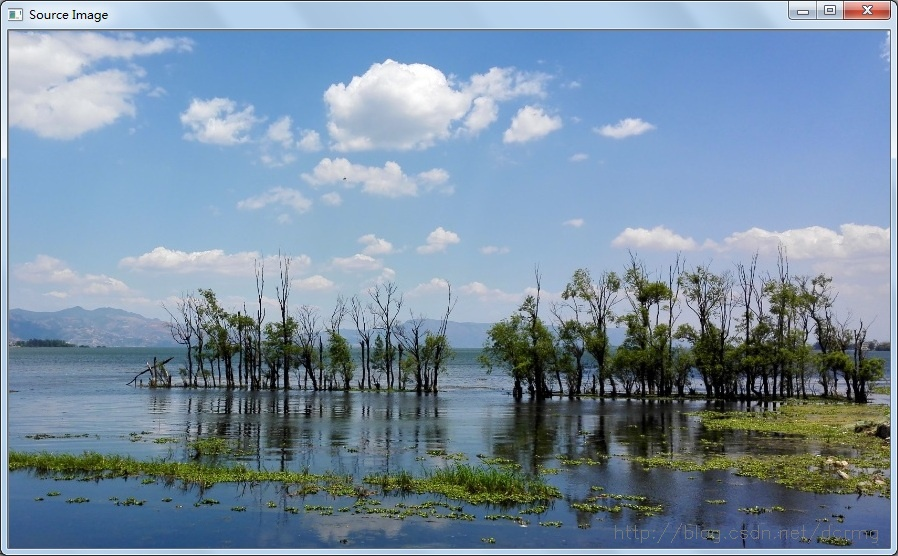卷积核尺寸5*5，σ=0.1：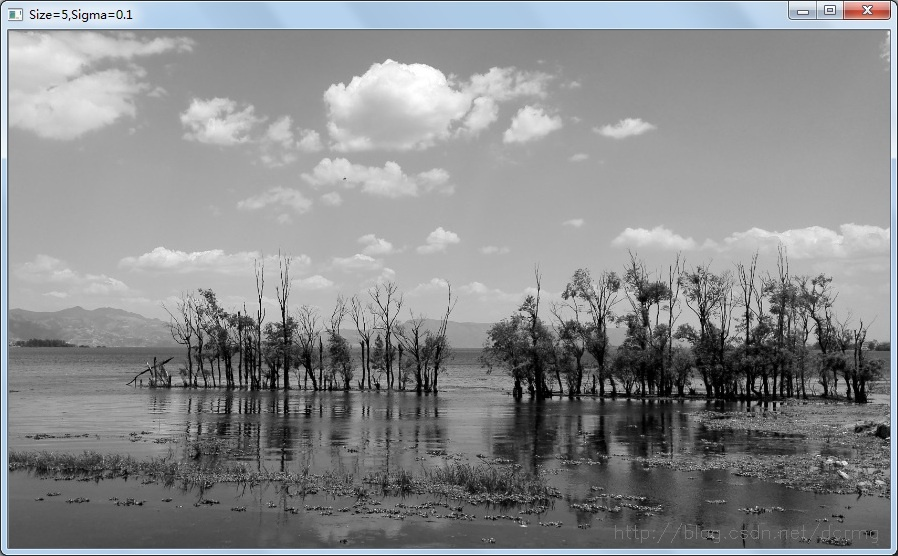卷积核尺寸5*5，σ=1：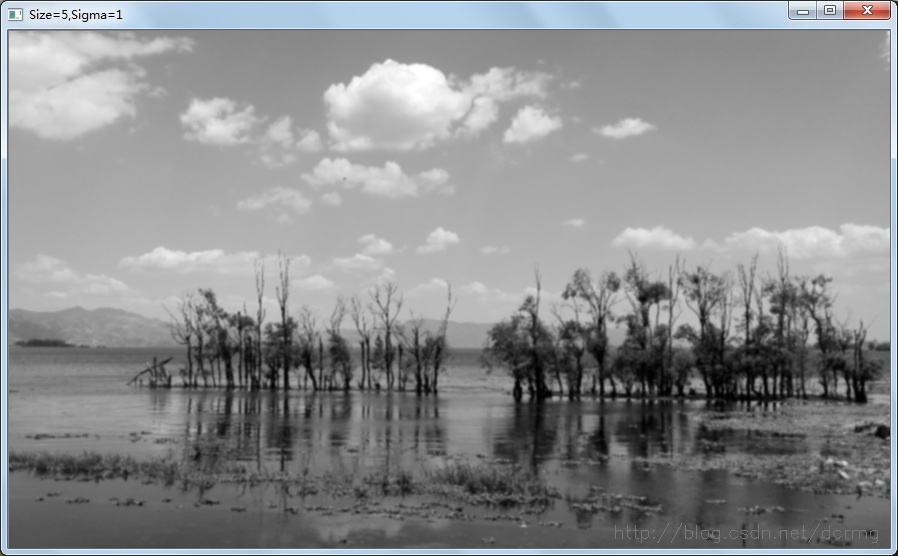对比可以看到，Sigma(σ)越大，平滑效果越明显。

C++对高斯卷积核参数求解：

[cpp]
view plain
copy

#include "iostream"   #include "math.h"      using namespace std;    using namespace cv;        //******************高斯卷积核生成函数*************************   //第一个参数gaus是一个指向含有3个double类型数组的指针；   //第二个参数size是高斯卷积核的尺寸大小；   //第三个参数sigma是卷积核的标准差   //*************************************************************   void GetGaussianKernel(double **gaus, const int size,const double sigma);      int main(int argc,char *argv[])     {       int size=5; //定义卷积核大小       double **gaus=new double *[size];       for(int i=0;i<size;i++)       {           gaus[i]=new double[size];  //动态生成矩阵       }       cout<<"尺寸 = 3*3，Sigma = 1，高斯卷积核参数为："<<endl;       GetGaussianKernel(gaus,3,1); //生成3*3 大小高斯卷积核，Sigma=1；          cout<<"尺寸 = 5*5，Sigma = 10，高斯卷积核参数为："<<endl;       GetGaussianKernel(gaus,5,10); //生成5*5 大小高斯卷积核，Sigma=1；         system("pause");       return 0;   }      //******************高斯卷积核生成函数*************************   void GetGaussianKernel(double **gaus, const int size,const double sigma)   {       const double PI=4.0*atan(1.0); //圆周率π赋值       int center=size/2;       double sum=0;       for(int i=0;i<size;i++)       {           for(int j=0;j<size;j++)           {               gaus[i][j]=(1/(2*PI*sigma*sigma))*exp(-((i-center)*(i-center)+(j-center)*(j-center))/(2*sigma*sigma));               sum+=gaus[i][j];           }       }          for(int i=0;i<size;i++)       {           for(int j=0;j<size;j++)           {               gaus[i][j]/=sum;               cout<<gaus[i][j]<<"  ";           }           cout<<endl<<endl;       }       return ;   }

求得的高斯卷积核参数存放在一个大小为N*N的数组内：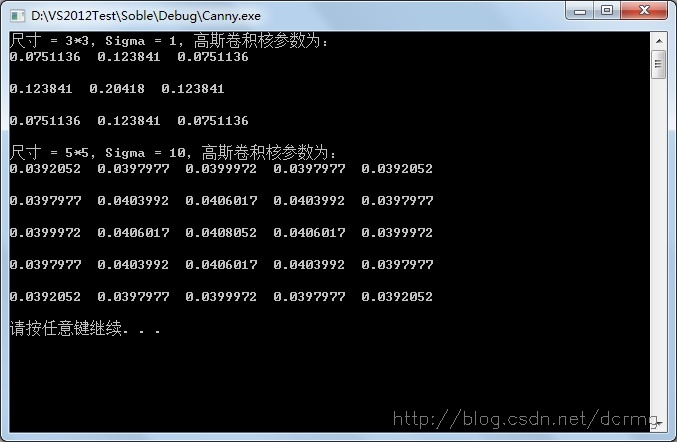展开全文• plt.imshow(median_blur_img) plt.subplot(1,4,4) plt.title("gaussian blur image") plt.imshow(img) plt.show() 锐化滤波主要是检测计算图像梯度，主要存在两种梯度，一种是一阶微分，使用图像像素的一阶差分可以...视觉检测 深度学习 自动驾驶 神经网络 数据挖掘
• 双边滤波器、高斯滤波 双边滤波（Bilateral filter）是一种非线性的滤波方法，是结合图像的空间邻近度和像素值相似度的一种折衷处理，同时考虑空域信息和灰度相似性，达到保边去噪的目的。普通的高斯滤波会将图像的...
• ## 高斯滤波

千次阅读 2017-05-15 20:13:03
图像滤波高斯滤波（Gauss filter） 概述： 高斯滤波高斯滤波在图像处理概念下，将图像频域处理和时域处理相联系，作为低通滤波器使用，可以将低频能量（比如噪声）滤去，起到图像平滑作用。opencv 图像处理
• 概率卡尔曼滤波优化更新(扩散卡尔曼滤波)， 正则化深度图； 3. 全局地图优化 Map Optimization， 关键帧加入当地图，从地图中匹配最相似的关键帧，估计sim3位姿变换，LM优化最小化变换误差. G2O图优化 ===...
• 高斯差分算子（Difference of Gaussian，DoG） 高斯差分算子（Difference of Gaussian，DoG）提取图像f(x,y)f(x, y)f(x,y)边缘：使用两个高斯低通滤波器分别平滑图像，然后与二者差值 高斯低通滤波（a convolution ...
• 卷积定理 函数空间域的卷积的傅里叶变换是函数傅里叶变换的乘积。对应地，频率域的卷积与空间域的乘积存在对应关系。...小的滤波模板以提高滤波速度。因为相同尺寸下，频域滤波器效率高于空域滤波器，故空域...
• ## 高斯噪声与高斯滤波

万次阅读 多人点赞 2017-10-09 12:06:58
噪声噪声表现形式噪声在图像上常表现为一引起较强视觉效果的孤立像素点或像素块。一般，噪声信号与要研究的对象不相关，它以无用的信息形式出现，扰乱图像的可观测信息。通俗的说就是噪声让图像不清楚。...高斯
• DOG高斯差分实现物体识别中的特征提取,对不同尺度空间的图像进行差分，从而获得描述物体的特征值
• 文章目录高斯滤波的理解与学习目录前言高斯函数一维高斯函数二维高斯函数高斯滤波过程高斯核求解利用高斯滤波高斯滤波步骤高斯滤波实现高斯滤波标准与窗口大小的换算实现常规实现分离实现高斯滤波总结高斯函数...计算机视觉 图像处理 人工智能
• ## matlab实现图像滤波——高斯滤波

万次阅读 多人点赞 2016-10-07 14:49:44
生成一个（2N+1）×（2N+1）大小的高斯模板H（标准为sigma），然后用此模板对图像进行滤波。不允许使用 fspecial 来产生高斯模板，不允许使用 imfilter、conv2 等函数。 原理及算法 理解：高斯滤波就是对整幅...matlab 数字图像
• ## 高斯差分

千次阅读 2020-01-13 20:05:16
DOG（Difference of Guassian）：简称 高斯函数的差分，是灰度图像增强和角点检测的一种方法。 （一）理论基础： 下面详细介绍DOG的角点检测（也称特征点提取）的理论过程： 通过将目标图像与高斯函数进行卷积...
• 高斯平滑滤波器SYxxxxxxx XX一、实验名称：实现高斯平滑滤波器选择几个不同的 ( 至少5个)对一幅图像进行滤波，观测不同的值对图像的平滑程度(注意 取值与窗函数大小的关系)。并说明如何为一幅图像选择合适的 值。二...
• ## matlab实现图像的高斯滤波

万次阅读 多人点赞 2019-04-23 16:09:08
高斯滤波的含义：高斯滤波就是对整幅图像进行加权平均的过程，每一个像素点的值，都由其本身和邻域内的其他像素值经过加权平均后得到。 高斯滤波的作用：高斯滤波是一种线性平滑滤波，适用于消除高斯...二维高斯分...
• 图像平滑处理（归一化块滤波高斯滤波、中值滤波、双边滤波） 2014-03-22 20:30 14961人阅读 评论(0) 收藏 举报  分类： 图像预处理（4）  版权声明：本文为博主原创文章，未经博主...
• 一、图像处理之均值滤波高斯滤波(高斯模糊)，中值滤波，双边滤波 滤波器介绍 滤波器作为图像处理课程的重要内容，大致可分为两类，空域滤波器和频率域滤波器。本文主要介绍常用的四种滤波器：中值滤波器、均值...
• 人类的世界就是一个信号传输的世界，所以噪声无处不在，图像作为一种信号传输的方式当然也无法幸免。为了尽量减少噪声对图像质量的影响，还原...然后就大致位空域滤波、变换域滤波和时域滤波，当然还有一些其他方式算法 自动驾驶 matlab
• 高斯滤波 高斯滤波器是一类根据高斯函数的形状来选择权值的线性平滑滤波器。高斯平滑滤波器对于抑制服从正态分布的噪声非常有效。一维零均值高斯函数为： g(x)=exp(-x^2/(2sigma^2)opencv定位
• 任务：用高斯滤波对图像进行降噪。 Step1：高斯滤波是啥？ 高斯滤波器是用于图像平滑处理的一种手段，性质为线性平滑，可对噪声进行过滤，达到降噪的目的。高斯滤波在数学上的体现就是对整个图像像素值通过加权...
• * @brief gaussianBlur 高斯滤波 * @param src 輸入可以為多通道圖，會單獨處理各通道，但是通常使用單通道灰階圖，例如CV_8U或CV_16U。 * @param dst 输出图 * @param ksize 模板大小，長寬可以不同，但是都必......